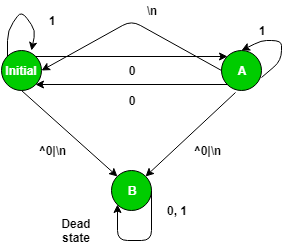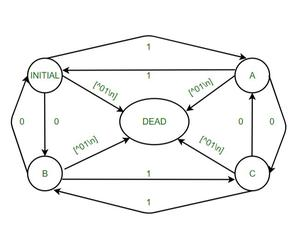# DFA in LEX code which accepts even number of zeros and even number of ones

• Difficulty Level : Medium
• Last Updated : 17 Dec, 2021

Lex is a computer program that generates lexical analyzers, which is commonly used with the YACC parser generator. Lex, originally written by Mike Lesk and Eric Schmidt and described in 1975, is the standard lexical analyzer generator on many Unix systems, and an equivalent tool is specified as part of the POSIX standard. Lex reads an input stream specifying the lexical analyzer and outputs source code implementing the lexer in the C programming language.

Deterministic Finite Acceptor –
In the theory of computation, a branch of theoretical computer science, a deterministic finite automaton (DFA)— also known as a deterministic finite acceptor (DFA) and a deterministic finite state machine (DFSM)— is a finite-state machine that accepts and rejects strings of symbols and only produces a unique computation (or run) of the automaton for each input string. Deterministic refers to the uniqueness of the computation. In search of the simplest models to capture finite-state machines, McCulloch and Pitts were among the first researchers to introduce a concept similar to finite automata in 1943.

Approach –LEX provides us with an INITIAL state by default. So to make a DFA, use this initial state as the initial state of the DFA. Define two more states A and B where B is the dead state that would be used if encounter a wrong or invalid input. When the user gets input that is invalid input, move to state B and the print message “INVALID” and if the user reaches INITIAL state from state A with a “\n” then display a message “Not Accepted”. But if the user get a \n on the initial state, the user display a message “Accepted”.

Examples –

```Input : 1001
Output : Accepted

Input : hjabdba
Output : INVALID```

To implement the above DFA, the user needs to write the below code in a lex file with a .l Extension.

NOTE :

To compile a lex program, user need a UNIX system and flex which can be installed using sudo apt-get install flex
With all the above specification open unix terminal and do the following:
1. Use the lex program to change the specification file into a C language program. The resulting program is in the lex.yy.c file.
2. Use the cc command with the -ll flag to compile and link the program with a library of lex subroutines. The resulting executable program is in the a.out file.

```lex lextest
cc lex.yy.c -lfl```

Code –

Output –

```nickhil@NICKHIL:~\$ lex prpg11.l
nickhil@NICKHIL:~\$ cc lex.yy.c -lfl
nickhil@NICKHIL:~\$ ./a.out
1000
Not Accepted
hello
INVALID
01010101
Accepted```

METHOD 2:-

Approach:-

LEX provides us with an INITIAL state by default. So to make a DFA, use this as the initial state of the DFA. We define four more states: A, B, C, and DEAD where DEAD state would be used if encountering, a wrong or invalid input. When user input an invalid character, move to DEAD state and print message “INVALID” and if input string ends at state INITIAL then display a message “Accepted”. If input string ends at state A, B, C then display a message “Not Accepted”.LEX CODE:-

```%{

%}

%%

<INITIAL>1 BEGIN A;

<INITIAL>0 BEGIN B;

<INITIAL>\n BEGIN INITIAL; {printf("Accepted\n");}

<A>1 BEGIN INITIAL;

<A>0 BEGIN C;

<A>\n BEGIN INITIAL; {printf("Not Accepted\n");}

<B>1 BEGIN C;

<B>0 BEGIN INITIAL;

<B>\n BEGIN INITIAL; {printf("Not Accepted\n");}

<C>1 BEGIN B;

<C>0 BEGIN A;

<C>\n BEGIN INITIAL; {printf("Not Accepted\n");}

%%

int main()

{

printf("Enter String\n");

yylex();

return 0;

}```

OUTPUT:-

```kashyap@kashyap-singh:~\$ lex e0e1.l
Kashyap@Kashyap-singh:~\$ cc lex.yy.c -lfl
Kashyap@Kashyap-singh:~\$ ./a.out
1010
Accepted
hello
INVALID
11100
Not Accepted
111100
Accepted
0001
Not Accepted```

My Personal Notes arrow_drop_up